Ask your WordPress questions! Pay money and get answers fast!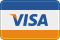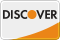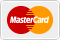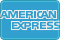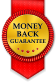# GravityForms dynamic radio buttons from custom post WordPress

• REFUNDED

Looking to have a GravityForms radio button entry dynamically populated using a dynamically created variable.

I have the following code:
``` //Adds a filter to form id 1. add_filter("gform_pre_render_1", "populate_radio_14"); add_filter("gform_admin_pre_render_1", "populate_radio_14"); function populate_radio_14(\$form){ \$coupon_book_5x = do_shortcode('[coupon_voucher_5x]'); \$coupon_book_10x = do_shortcode('[coupon_voucher_10x]'); //Creating radio choices \$choices = array( array("text" => "Coupon Voucher 5x, \$\$coupon_book_5x.00", "value" => "5x_coupon_voucher"), array("text" => "Coupon Voucher 10x, \$\$coupon_book_10x.00", "value" => "10x_coupon_voucher") ); /* \$inputs = array( array("label" => "Coupon Voucher 5x", "id" => "14.0", "value" => "5x_coupon"), // field id array("label" => "Coupon Voucher 10x", "id" => "14.1", "value" => "10x_coupon") // field id );*/ //Adding items to field id 14. foreach(\$form['fields'] as &\$field){ if(\$field['id'] == 14){ \$field['choices'] = \$choices; // \$field['inputs'] = \$inputs; } } return \$form; } ```

It correctly renders the form in the front end but does not pass the variables after the form is submitted.

P.S. Yes I have checked out the following and have found them unhelpful.
http://www.gravityhelp.com/documentation/page/Using_Dynamic_Population
http://www.gravityhelp.com/documentation/page/Allow_field_to_be_populated_dynamically

``` The shortcodes are processed with this function: /** 4. Coupon Voucher 5x * @example <code>[coupon_voucher_5x]``` is the default usage */
function scn_coupon_voucher_5x(\$atts, \$content = null) {
\$coupon_voucher_5x = get_field('coupon_voucher_5x','option');
return \$coupon_voucher_5x;
} // End scn_coupon_voucher_5x()

/** 5. Coupon Voucher 10x
* @example `[coupon_voucher_10x]` is the default usage */
function scn_coupon_voucher_10x(\$atts, \$content = null) {
\$coupon_voucher_10x = get_field('coupon_voucher_10x','option');
return \$coupon_voucher_10x;
} // End scn_coupon_voucher_10x()

Basically, the coupon prices are defined using Advanced Custom Fields, then dumped into shortcodes, and used in the dynamic radio button code.

2014-07-16

You're showing the frontend code... where are you not seeing the variable? Is it in the entry object, form object, or post object?